This is for a simple roof with a max of 4 slopes.  You can use it for one slope, two slopes, three slopes or 4 slopes.  All boxes need to have a value, so if you are doing a simple gable roof then be sure to leave the 0 in the other slopes.

To use just enter your measures and click calculate at the bottom of the form. We will soon add another form for more complicated roofs.

 Slope 1 Height:
 Slope 1 Width:
 Area 1 Total:

 Slope 2 Height:
 Slope 2 Width:
 Area 2 Total:

 Slope 3 Height:
 Slope 3 Width:
 Slope 3 Total:

 Slope 4 Height:
 Slope 4 width:
 Slope 4 total:

 Waste Factor: Gable 10%, Hip 15%, Gambrel 10%, Mansard 15% 5% 10% 15% 20%
 Total Tear-off sf:
 Total replace square feet:
 Total Remove Sqs:
 Total Replacement Sqs: Be sure to round this amount up to the next full bundle
Calculate

CADOgrams™ Ready Made Roof Diagrams, are computerize diagrams that are free for you to use.  There are at least two ways to use the diagrams.
1.Select a diagram from the list below and click on it. You will then see a page with the diagram. All you need to do it fill in the blanks and use the  browsers print function to print the form out. Follow this link for a sample.

2. Go to the diagram form and right click on the photo and download it to your hard drive. You then can insert in a document and add your own text.

 1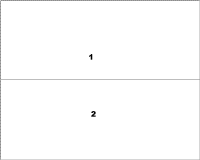2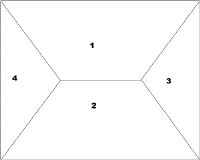34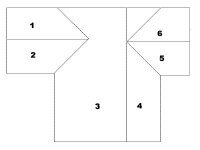5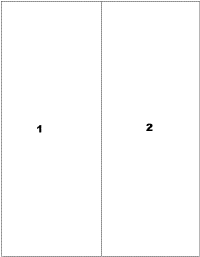67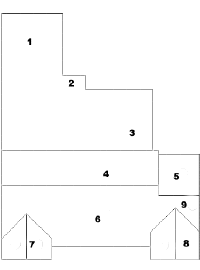8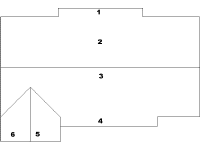9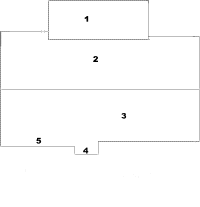10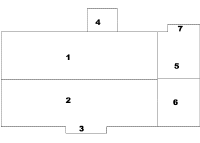11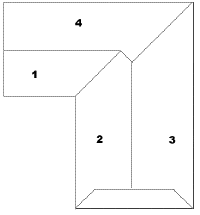121314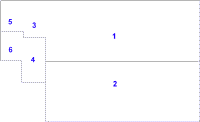This has been tested with IE 5.0 and Netscape 4.51 and found to work with both. These diagrams are diagrams of actual roofs that I have inspected.

1" = .08    2" = .17   3" = .25

4" = .33    5" = .42   6" = .50

7" = .58    8" = .67   9" =  .75

10" = .83   11" = .92  12" = 1.00

 Total Sqs: Enter the total squares
 Waste Factor: In the waste Factor 5% 10% 15% 20%
 Waste amount: This is in squares,
 Total replacment amount: This is the total, you need to round up to the nearest bundle.